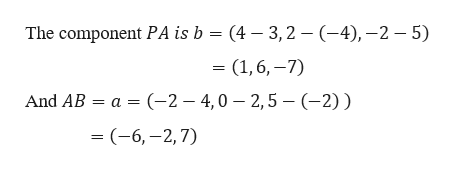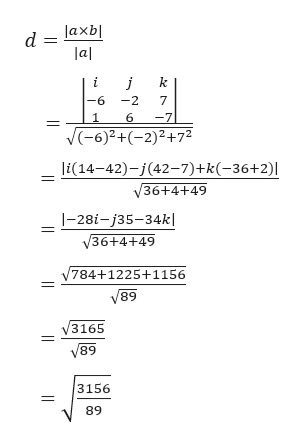Question
734 views
 The distance dd of a point P to the line through points A and B is the length of the component of AP that is orthogonal to AB, as indicated in the diagram. So the distance from P=(3,−4,5) to the line through the points A=(4,2,−2) and B=(−2,0,5) is
check_circle

star
star
star
star
star
1 Rating
Step 1

Given,

The distance d of a point P to the line through points A and B is the length of the component of AP that is orthogonal to AB, as indicated in the diagram.

Step 2

Here, we have P = (3, −4, 5), A = (4, 2, −2) and B = (−2, 0, 5).

Now,help_outlineImage TranscriptioncloseThe component PA is b = (4 - 3,2 (-4),-2 - 5) = (1,6,-7) And AB a (-2 - 4,0 2,5- (-2) ) - (-6,-2, 7) fullscreen
Step 3

Therefore, the distanc...help_outlineImage Transcriptionclosed laxb lal j k 6 2 7 6 -7 |(-6)2+(-2)2+72 li(14-42)-(42-7)+k(-36+2)| V36+4+49 |-28i-j35-34k| /36+4+49 /784+1225+1156 68 3165 V89 3156 89 fullscreen

### Want to see the full answer?

See Solution

#### Want to see this answer and more?

Solutions are written by subject experts who are available 24/7. Questions are typically answered within 1 hour.*

See Solution
*Response times may vary by subject and question.
Tagged in

### Other